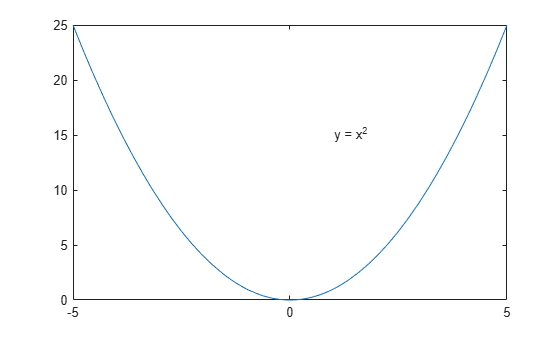Documentation

# texlabel

TeX representation of symbolic expression

## Syntax

texlabel(expr)
texlabel(expr,'literal')

## Description

example

texlabel(expr) converts the symbolic expression expr into the TeX equivalent for use in character vectors. texlabel converts Greek variable names, such as delta, into Greek letters. Annotation functions, such as title, xlabel, and text can use the TeX character vector as input. To obtain the LaTeX representation, use latex.

example

texlabel(expr,'literal') interprets Greek variable names literally.

## Examples

### Generate TeX Character Vector

Use texlabel to generate TeX character vectors for these symbolic expressions.

syms x y lambda12 delta texlabel(sin(x) + x^3) texlabel(3*(1-x)^2*exp(-(x^2) - (y+1)^2)) texlabel(lambda12^(3/2)/pi - pi*delta^(2/3))
ans = '{sin}({x}) + {x}^{3}' ans = '{3} {exp}(- ({y} + {1})^{2} - {x}^{2}) ({x} - {1})^{2}' ans = '{\lambda_{12}}^{{3}/{2}}/{\pi} - {\delta}^{{2}/{3}} {\pi}' 

To make texlabel interpret Greek variable names literally, include the argument 'literal'.

texlabel(lambda12,'literal')
ans = '{lambda12}'

### Insert TeX in Figure

Plot y = x^2 using fplot. Show the plotted expression y by using texlabel to generate a TeX character vector that text inserts into the figure.

syms x y = x^2; fplot(y) ylabel = texlabel(y); text(1, 15, ['y = ' ylabel]);## Input Arguments

collapse all

Expression to be converted, specified as a symbolic expression.